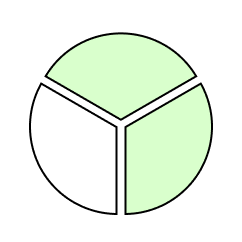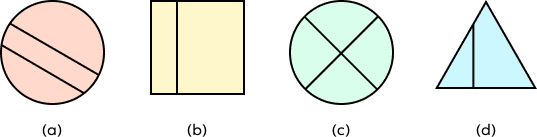# Unequal Parts in Math – Definition With Facts, Examples

Home » Math-Vocabluary » Unequal Parts in Math – Definition With Facts, Examples

## What Are Unequal Parts?

Unequal simply means “not equal.” So, what are unequal parts? When we cut a piece of paper into different shapes, not all pieces will be of the same size. When we distribute a pie or a cake into different pieces depending on everyone’s appetite, each piece will be of a different size.

Let us look at some examples.

Example 1: A cake divided into unequal parts or unequal shares.

Example 2: Square shape: equal and unequal parts

## Unequal Parts: Definition

Two parts of a whole are said to be unequal when they do not match each other in terms of their sizes and values.

“Unequal” definition in math: Unequal means “not equal” in size, shape, value, or any other aspect.

Let us look at an example of unequal parts in shapes.

In the given image, we see that a circle is divided into 4 parts. However, all the parts are not equal in shape and size.

## Understanding Unequal Parts with Fractions

Fractions represent parts of a whole. For example, the fraction $\frac{2}{5}$ represents 2 parts out of 5 equal parts of a whole.

If a pizza is divided into 8 equal slices, each part is said to represent a fraction $\frac{1}{8}$.

Now, suppose we cut the cake into fractions $\frac{1}{8},\; \frac{2}{8},\; \frac{5}{8}$. Each slice will be a different size and we will get unequal parts of the pizza.

When we talk about fractions, two fractions are said to be unequal to each other if their lowest forms are not equal to each other.

Consider the fractions $\frac{50}{100}$ and $\frac{30}{90}$.

On simplifying $\frac{50}{100}$, we get

$\frac{50}{100} \div \frac{50}{50} = \frac{1}{2}$

Similarly, on simplifying $\frac{30}{90}$ , we get

$\frac{30}{90} \div \frac{30}{30} = \frac{1}{3}$

We observe that the simplest forms of $\frac{50}{100}$ and $\frac{30}{90}$ are not equal. So, the fractions are not equal. In other words,

• Unequal parts refer to divisions or sections that are not equal in size or quantity.
• Understanding equal parts and unequal parts helps develop a sense of fairness and equality, as children learn to recognize and compare different portions or shares.
• Not equal sign is given as $\neq$.It is used to represent two quantities that are not equal in amount, or value.

## Conclusion

In this article, we learned about unequal parts along with diagrams. We also saw daily instances where we see unequal parts. We can now study unequal fractions with some examples.

## Solved Examples on “Unequal Parts”

1.  Find the images representing unequal parts.

Solution:

Images that represent unequal parts are image (a) and image (b).

In the image (c), the four parts are equal.

1. Does the dotted line divide the star into equal or unequal parts?

Solution:

The dotted line is dividing the star into equal parts. The two parts are identical and will match perfectly if placed on one another.

1. Are the fractions $\frac{1}{8}$ and $\frac{2}{4}$ equal?

Solution:

$\frac{1}{8}$ is in its lowest form.

Simplifying $\frac{2}{4}$, we get

$\frac{2}{4} = \frac{1}{2}$

The simplified/lowest forms of $\frac{1}{8}$ and $\frac{2}{4}$ are not equal. So, the fractions are not equal.

4. Is the given triangle divided into equal or unequal parts?

Solution:

The triangle is divided into 4 parts and all the parts are of different sizes.

So, they represent unequal parts.

5. Are the given fractions equal in parts?

$\frac{80}{100},\; \frac{60}{12}$

Solution:

Simplify $\frac{80}{100}$.

$\frac{80}{100} \div \frac{20}{20} = \frac{4}{5}$

Simplify $\frac{60}{12}$.

$\frac{60}{12} \div \frac{12}{12} = \frac{5}{1}$

Hence, fractions are not equal in parts.

## Practice Problems on “Unequal Parts”

1

### Which of the given fractions are equal to $\frac{20}{25}$?

$\frac{18}{100}$
$\frac{45}{200}$
$\frac{9}{20}$
$\frac{4}{5}$
CorrectIncorrect
Correct answer is: $\frac{4}{5}$
Simplify $\frac{20}{25}$. $\frac{20}{25} \div \frac{5}{5} = \frac{4}{5}$
2

### What do we get on dividing the number 891 into 3 equal parts?

81, 81, 81
97, 97, 97
297, 297, 297
351, 351, 351
CorrectIncorrect
Correct answer is: 297, 297, 297
If we divide the number 891 by 3, we get
$\frac{891}{3} = 297$
So, three equal parts are (297, 297, 297).
3

### What is the fraction represented by the green part?$\frac{1}{9}$
$\frac{1}{3}$
$\frac{2}{3}$
$\frac{1}{6}$
CorrectIncorrect
Correct answer is: $\frac{2}{3}$
The circle is divided into 3 equal parts. Each part represents $\frac{1}{3}. Two such parts make the orange shaded region. So, they represent$\frac{2}{3}$. 4 ### Which image shows equal parts?Image (a) Image (b) Image (c) Image (d) CorrectIncorrect Correct answer is: Image (c) The circle is divided into 4 equal parts. The rest of the options show unequal parts. 5 ### Identify the image which shows equal parts.Image (a) Image (b) Image (c) Image (d) CorrectIncorrect Correct answer is: Image (c) Option c) shows two identical halves of the heart shape. ## Frequently Asked Questionson “Unequal Parts” When we divide an object/number into two equal parts, each new individual part is known as half. They are collectively known as halves. When we divide an object/number into four equal parts, each new individual part is known as a quarter. They collectively are known as quarters. In mathematics, especially in geometry, an object is said to have symmetry if it can be divided into two identical halves. Fractions in which numerators and denominators are different, but still represent the same value are called equivalent fractions. Example:$\frac{1}{2}$and$\frac{2}{4}\$

Any object or shape that can be cut in two identical halves or two equal parts is called a symmetrical object.